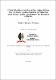# Contribution to iterative algorithms for certain optimization problems and fixed-point problems in Banach spaces.2018

## Abstract

We study the convergence analysis of the xed points set of common solution of a one- parameter nonexpansive semigroup, the set of solution of constrained convex minimization problem and the set of solutions of generalized equilibrium problem in a real Hilbert space using the idea of regularized gradient-projection algorithm. Also, we look at the strong convergence of a modi ed gradient projection algorithm and forward-backward algorithm in Hilbert spaces with numerical computations. We also introduce an iterative algorithm for approximating a common solution of generalized mixed equilibrium problem and xed point problem in a real re exive Banach space. Using our algorithm, a strong convergence theorem is proved concerning an element in the intersection of set of solutions of general- ized mixed equilibrium problem and the set of solutions of xed point for a nite family of Bregman strongly nonexpansive mappings. Moreover, we study and analyze an iterative method for nding a common element of the xed points set of an in nite family of k-demicontractive mappings which is also a solution to a zero of the sum of two monotone operators, with one operator being maximal monotone and the other inverse-strongly monotone. We further extend our study from the frame work of real Hilbert spaces to more general real smooth and uniformly convex Banach spaces. In this space, we introduce an iterative algorithm with Meir-Keeler contractions for nding zeros of the sum of nite families of m-accretive operators and nite family of inverse strongly accretive operators. We apply our result to the approximation of solution of certain integro-di erential equation with generalized p-Laplacian operators. Furthermore, we study the convergence theorem for a new class of split variational inequal- ity and variational inclusion problem in Hilbert space. We further considered split equality for minimization problem and xed point sets, split xed point problem and monotone inclusion problems, split equilibrium problem and xed point set for multivalued map- pings. All these of our algorithms involve a step-size selected in such a way that their implementation does not require the computation or an estimate of the spectral radius. Again, an iterative algorithm that does not require any knowledge of the operator norm for approximating a solution of split equality equilibrium and xed point problems in the frame work of p-uniformly convex Banach spaces which are also uniformly smooth is introduced of which we studied the approximation of solution of split equality generalized mixed equilibrium problem and xed point problem for right Bregman strongly quasi- nonexpansive mappings in q-uniformly convex Banach spaces which are also uniformly smooth. We also study and analyze an iterative algorithm for nding a common element of the set of the split equality for monotone inclusion problem and xed point of a right Bregman strongly nonexpansive mapping T in the setting of p-uniformly convex uniformly smooth Banach spaces. Finally, we present numerical examples of our theorems and apply our results to study the convex minimization problems and equilibrium problems.

## Description

Doctoral Degree. University of KwaZulu-Natal, Durban.